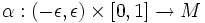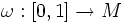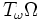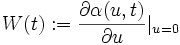# Variation vector field

Let$M$ be a differential manifold.
Let$\alpha: (-\epsilon,\epsilon) \times [0,1] \to M$ be a piecewise smooth variation of a curve$\omega:[0,1] \to M$. The variation vector field of$\alpha$ is defined as the following element of the tangent space$T_\omega\Omega$:$W(t) := \frac{\partial \alpha(u,t)}{\partial u}|_{u=0}$